# 1 Background

Prior course work introduced the concepts of Type I and II errors in some detail, but in a limited context. The Type II error concept is consistently defined across NHST methods as the probability of failing to reject the null hypothesis when it is false (and a spcecific alternative hypothesis is specified). This document does several things. First, it reviews, with simulation, the earlier classroom development of the Type II error concept in the context of a one-sample location test where the population variance is known. Then the document extends this idea to a one sample “t-tests” where population variances are unknown and are estimated. That sets the stage for further extension to other methods later in the course sequence. The most important additional concept that requires development is that of the non-centrality paramater and non-central distributions such as the non-central t. The approach is anchored in simulation rather than mathematical derivation, and it is facilitated by visualizations.

An additional goal of the document is the consolidation of a general understanding that can permit the student to use such tools as the pwr package in R or the GPower software that is widely used. Both can be important tools for sample size planning, but require a modicum of understanding of effect sizes, non-central distributions, and power.

# 2 R Essentials

Several R packages are required in this document. Theggplot2 package is used in multiple places and is available as a dependency with the ggfortify package.

library(psych)
library(car)
library(ggfortify)
library(knitr)
library(gt)
library(pwr)

Package citations for packages loaded here (in the above order): psych (Revelle, 2019), car (Fox, Weisberg, & Price, 2018), ggfortify (Horikoshi & Tang, 2019), ggplot2 (Wickham et al., 2018), knitr (Xie, 2018), gt (Iannone, Cheng, & Schloerke, 2019), pwr(Champely, 2018)

# 3 Review of power concepts using the ZM NHST method

The introduction to traditional null hypothesis significance testing is most readly accomplished with the single sample “Z of a mean” test. The null hypothesis specifies the mean ($${{\mu }_{o}}$$) of a distribution. The alternative typically specifies that the true mean ($${{\mu }_{1}}$$)is either different than the null value or deviant higher or lower when the test is a one tailed test. The test is called a “Z of a mean” test because the observed sample mean is standardized, relative to the null value

$$\left( \bar{X}-{{\mu }_{0}} \right)/\left( {{\sigma }_{X}}/\sqrt{n} \right)$$.

The standard error in the denominator is the theoretical std deviation of the sampling distribution of the mean, based on the population RV std deviation and sample size ($${}^{{{\sigma }_{x}}}/{}_{\sqrt{n}}$$). Our interest is in how this sample mean behaves (if repeated samples are taken from that null distribution) and we can rescale the sample mean in terms of std error units when we compute the “Z of a mean”

This Z of a mean taken on our one real sample is also called our test statistic. We compare it to values expected if the null is true by using a standard normal distribution. This document explores how means and test statistics behave under various hypotheses and other specifications. The goals are:

• furthering understanding of Type II errors.

• reinforcing the understanding that power is computed “against a specific alternative”.

• laying the foundation for consideration of non-central versions of the t, chi-square and F distributions.

## 3.1 Visualizing sampling distributions under different hypotheses. Part 1: The null hypothesis distribution.

First, let’s specify that sample(s) are drawn from a hypothetical population distribution of the RV where $${{\mu }_{0}}=100$$ (the null hypothesis value) and $${{\sigma }_{x}}=15$$ (a common occurence for many normed test instruments). Further assume that our samples are each comprised of 25 replications or observations (n=25). The theoretical sampling distribution of the mean based on these specifications has a standard deviation of 3 $$\left( {}^{{{\sigma }_{x}}}/{}_{\sqrt{n}} \right)$$.

We can simulate this by randomly drawing 10000 sample means from this sampling distribution -

($${{\mu }_{0}}=100$$ and $${{\sigma }_{x}}=15$$)

so, $$\left( {{\mu }_{{\bar{X}}}}=100,\,\,and\,\,{{\sigma }_{{\bar{X}}}}=3 \right)$$ when H0 is true.

#library(psych)
set.seed= 1000
means0 <- rnorm(10000,mean=100,sd=3)
headTail(means0) # shows the first few and last few elements of the object
##   [,1]               [,2]               [,3]               [,4]
## h "103.210547419981" "98.7801453293904" "105.224609483728" "98.910608775084"
##   "...       ..."    "...       ..."    "...       ..."    "...       ..."
## t "97.6338212696976" "106.50699597112"  "101.649283729742" "101.613405433688"

Next, we can draw a frequency histogram of those 10,000 means.

hist(means0,breaks=30, prob="TRUE")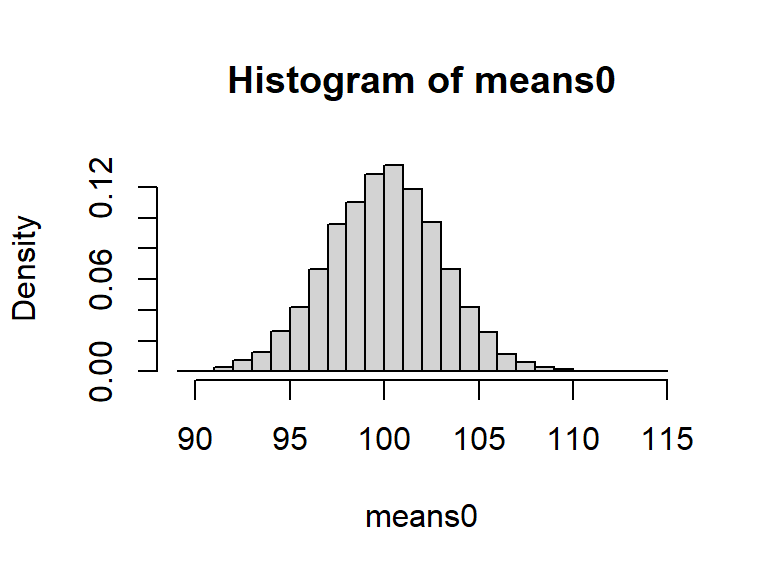And we can add a kernel density function to better visualize the shape.

hist(means0,breaks=30, prob="TRUE")
lines(density(means0,bw=1),col="red",lwd=2)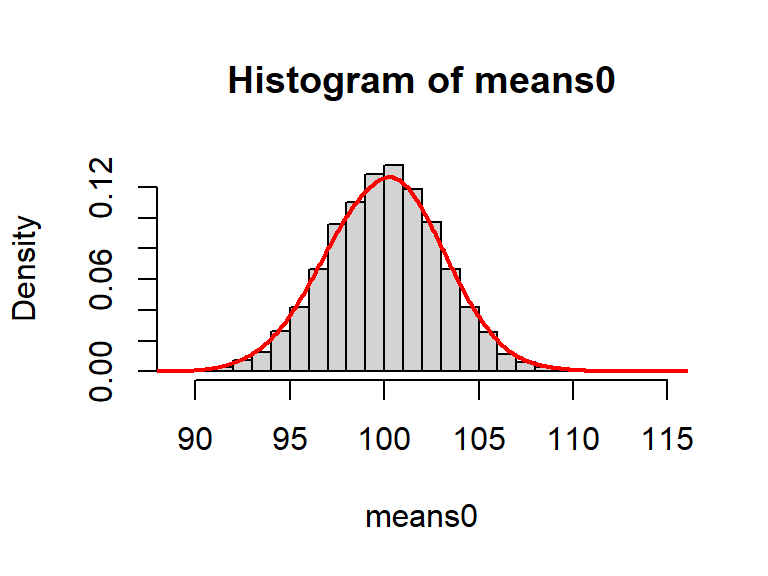This simulated distribution looks quite normal. Lets check with a qq plot.

#library(car)
qqPlot(means0) # the default qqPlot is a qq normal plot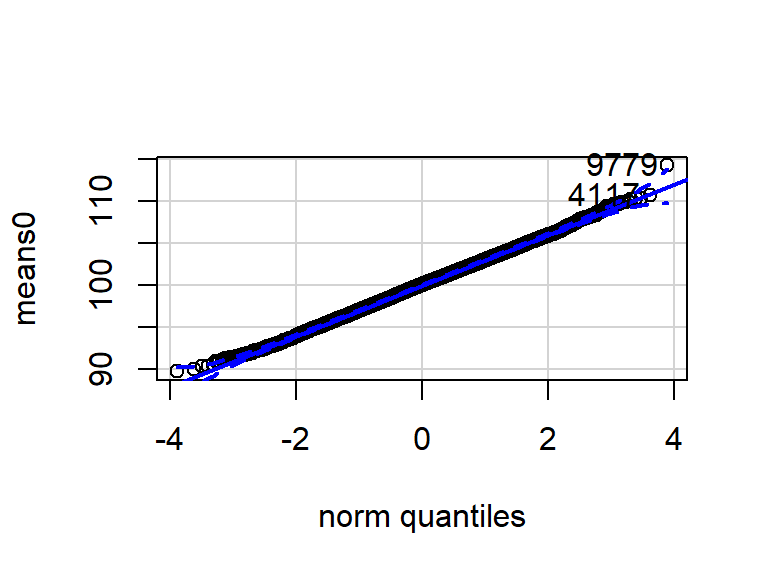##  9779 4117

What are the mean and std deviation of these simulated means? Are they close to the 100 and 3 values expected?

mean(means0)
##  100.0088
sd(means0)
##  3.009895

We can thus see how the $$\bar{X}$$ statistic “behaves” when the null is true and samples are drawn from that null hypothesis distribution.

But what if we converted our 10,000 means each to a “Z of a mean”? We need to subtract 100 from each and divide by the std error (3). We can do that operation with the whole vector of 10000 simulated means.

Since we are doing this with the sample means drawn from the null hypothesis distribution, we are simply doing a standardization of that sampling distribution. Assuming normality of the original sampling distribution, this distribution should also approximate a std normal.

zm0 <- (means0-100)/3
headTail(zm0)
##   [,1]                 [,2]                 [,3]
## h "1.07018247332702"   "-0.406618223536531" "1.74153649457611"
##   "...       ..."      "...       ..."      "...       ..."
## t "-0.788726243434141" "2.16899865703985"   "0.549761243247204"
##   [,4]
## h "-0.363130408305343"
##   "...       ..."
## t "0.537801811229253"

And we can visualize this standardized distribution in the same ways.

hist(zm0,breaks=30,prob="T")
lines(density(zm0,bw=.2),col="red",lwd=2)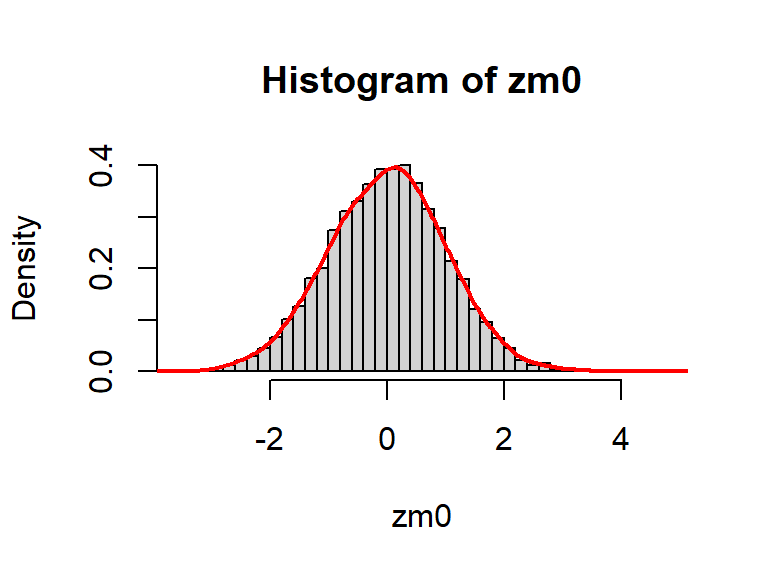It also shows the expected normality.

qqPlot(zm0)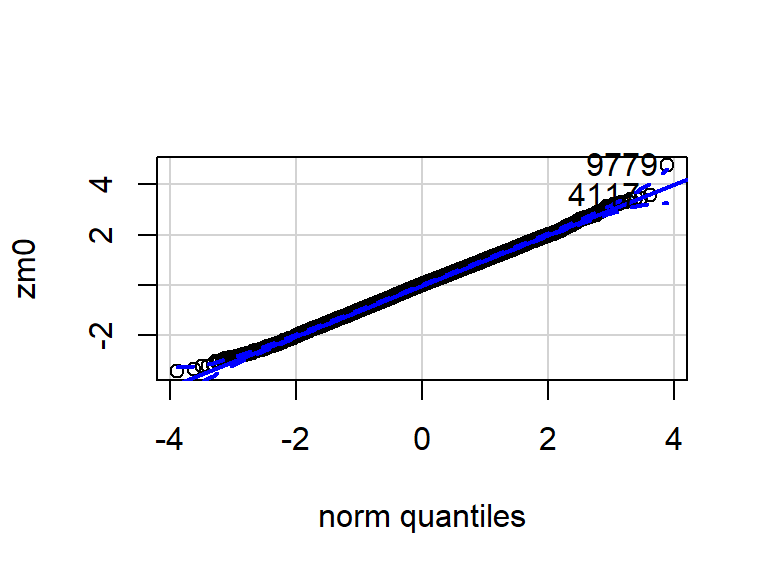##  9779 4117

The central idea here is that when a. the null is true and b. we take one sample, compute a mean, and standardize it, that Z of a mean can be viewed as coming from a standard normal Z distribution. Therefore, our TEST STATISTIC behaves in this specified way when the null is true (and the normality assumption is satisfied)

If we draw this theoretical null hypothesis distribution (the one that we just simulated) using ‘ggplot2’ tools we can also identify a “critical value” for a one-tailed (upper tail) test based on an alpha of .05 - thus “seeing” the Type I error rate. The first plot of this distribution uses the’ggdistribution’ function which makes the plot easy to draw, but does not permit some additional attributes that are found on the second plot. The ‘ggdistribution’ function can plot a distribution using any of the built-in probability functions that R has by using the “d” prefix for density.

# library(ggfortify)
p <- ggdistribution(dnorm, seq(-4, 4, .002), fill = 'honeydew3')
p +     geom_vline(aes(xintercept=qnorm(.05,0,1,lower.tail=F)),
linetype="solid", size=1, colour="blue") +
theme_minimal()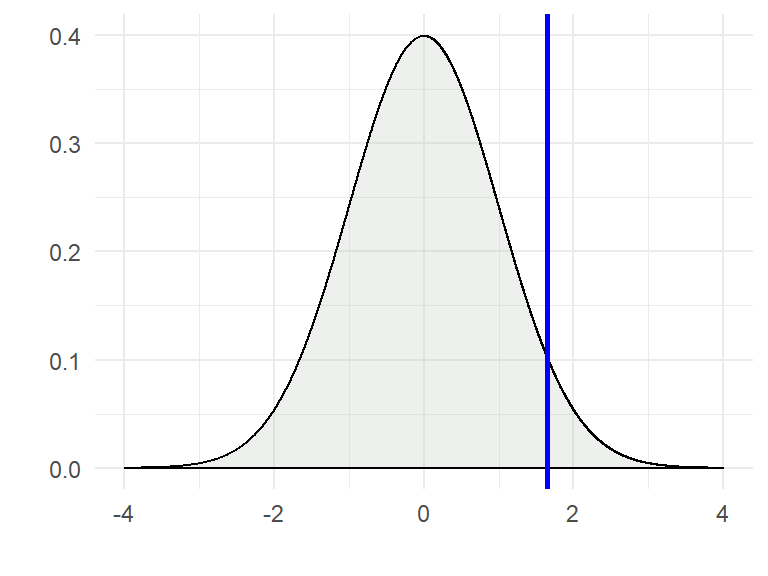The second way of doing this plot uses straight ‘ggplot2’ methods and controls many attributes of the plot.

#Plot prob dist in ggplot (not with ggfortify)
x <- seq(-4, 4, .002)
y <- dnorm(x,0,1)
dat <- cbind.data.frame(x,y)
p <- ggplot(dat, aes(x = x, y = y)) +
geom_line() +
geom_area(mapping = aes(x = ifelse(x>qnorm(.05,0,1,lower.tail=F), x, 0)), fill = "red") +
xlim(-4,4) + ylim(-.015,.4) +
geom_vline(aes(xintercept=qnorm(.05,0,1,lower.tail=F)),
linetype="solid", size=1, colour="blue") +
geom_hline(aes(yintercept=0),
linetype="solid", size=1, colour="black") +
annotate("text", label = "fail to reject", x = 0, y = -.01, size = 4, colour = "blue") +
annotate("text", label = "reject", x = 2.5, y = -.01, size = 4, colour = "red") +
labs(x="Z value", y="Density") +
theme_minimal()
p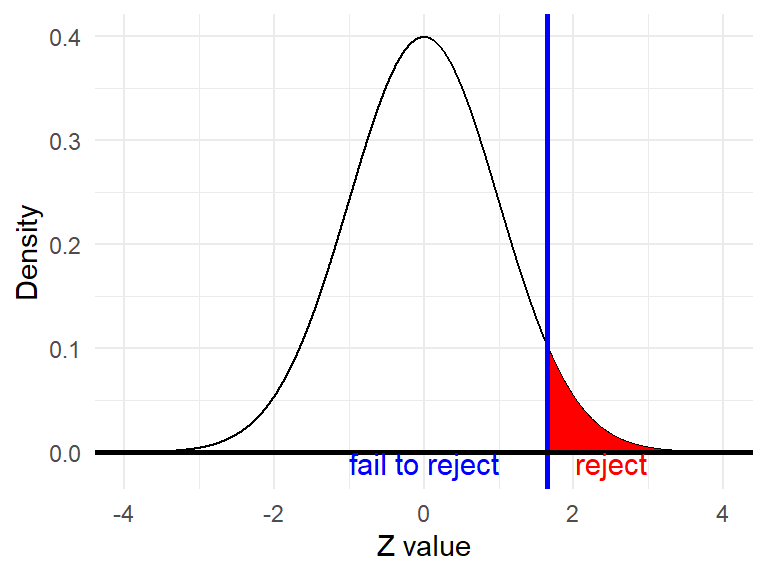## 3.2 Visualizing sampling distributions under different hypotheses. Part 2: The alternative hypothesis distribution.

If the null hypothesis is false, then means will not be distributed according to the specifications defined above for the first simulation. When the inferential test is performed, we typically specify “inexact” alternative hypotheses. But the understanding of Type II errors and power requires spcecification of an exact alternative. This does not change whether the test is done as a 1 or 2-tailed test with inexact alternative(s). We say that power and type II errors are found “against a specific alternative”. For example, if the true distribution that samples are drawn from has a mean higher than 100 (say 109), then the theoretical sampling distribution of the mean will be shifted nine units higher (or 3 standard errors). We can simulate this here.

set.seed= 1000
means1 <- rnorm(10000,mean=109,sd=3)
headTail(means1)
##   [,1]               [,2]               [,3]               [,4]
## h "110.389289228041" "112.349537983034" "106.045866893535" "108.569654899745"
##   "...       ..."    "...       ..."    "...       ..."    "...       ..."
## t "103.929726561564" "110.915483801034" "102.815398747204" "108.194967916122"

If we plot these simulated means in a histogram and add a density function, the shift to a location of 109 can be visualized, but note that the shape is still normal.

hist(means1,breaks=30, prob="TRUE")
lines(density(means1,bw=1),col="red",lwd=2)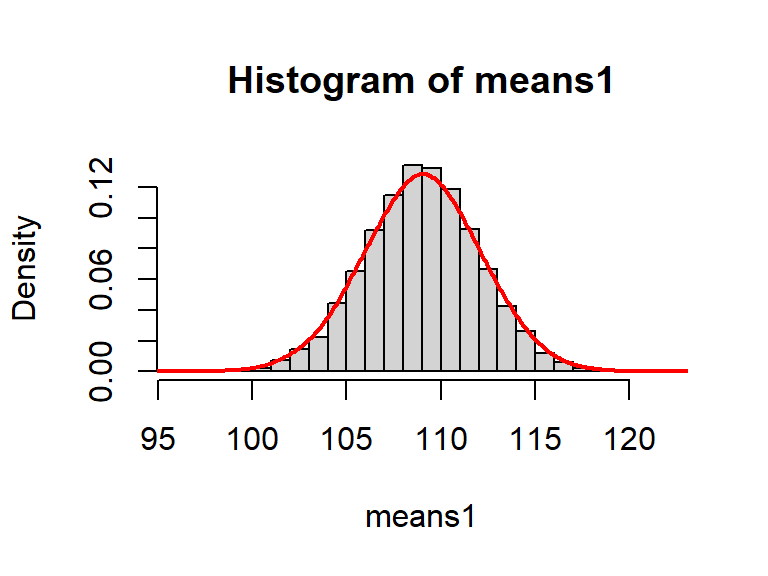If we standardize (relative to our null value of 100) our simulated set of means from this second simulation, we have another set of Zm’s. It’s shape is also normal, but note that the mean is at a Z value of approximately 3. This is because 109 is 3 standard errors above the null value that we standardized it to. This shift of 3 Z units can be described as an effect size (muo -mu1/std dev).

zm2 <- (means1-100)/3
mean(zm2)
##  3.000538
sd(zm2)
##  0.9990265

Plotting these standardized Zm values also reveals the expected normal shape (central limit theorem still in play).

hist(zm2,breaks=30, prob="TRUE")
lines(density(zm2, bw=.2),col="red",lwd=2)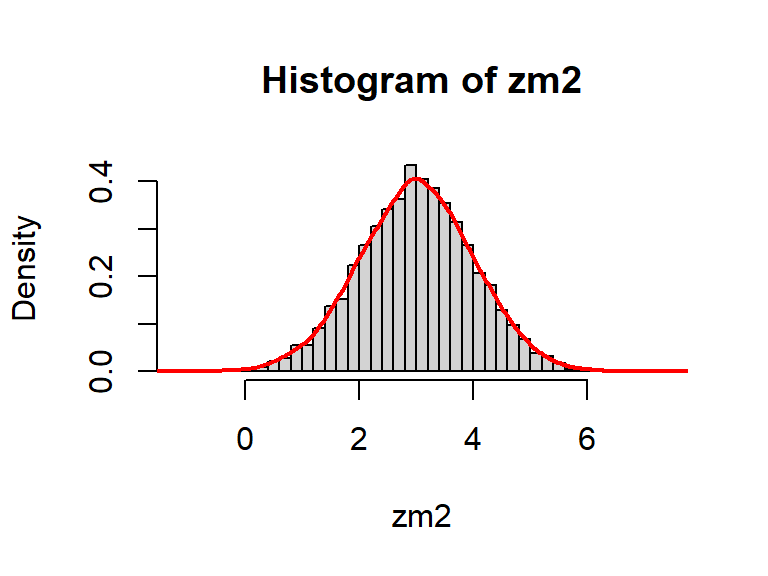Drawing a more accurate depiction of this theoretical sampling distribution with ggplot2 tools can reinforce the impression that the alternative hypothesis sampling distribution is normal.

p <- ggdistribution(dnorm, seq(-4, 8, .002), mean=3, sd=1, fill = 'honeydew2') +
theme_minimal()
p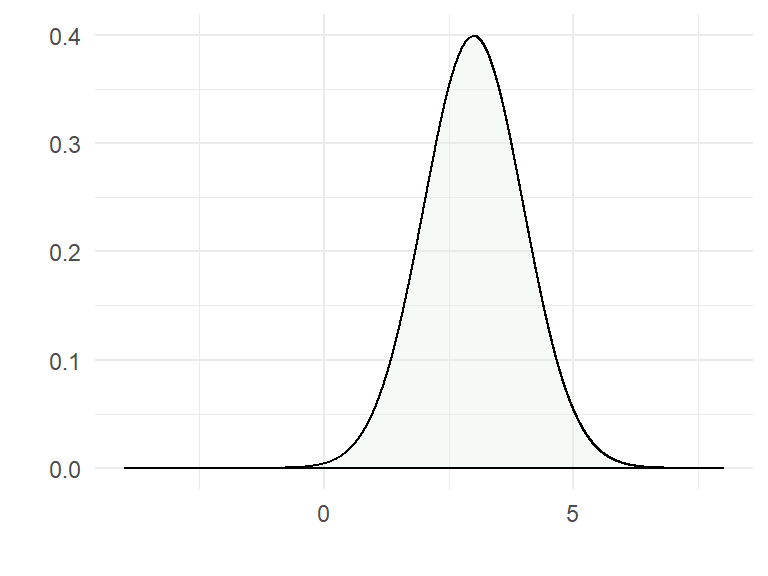## 3.3 Visualizing sampling distributions under different hypotheses. Part 3: Visualize both the null and alternative distributions

If we plot both of these theoretical sampling distributions of the Zm statistic on the same graph, and also include the visual indicator of the critical value (at the vertical line), then the familiar visualization of both alpha and beta/power is possible. Students will recognize the similarity of this visualization from the earlier introduction to this topic and with use of the SHINY app, except that the plots here are of the test statistic (ZM) rather than the raw sample means

The key elements are that 1) alpha is defined as an area based on the null distribution (it is .05) and 2) beta is found under the alternative distribution (it equals about .09). Another point to reinforce is that both sampling distributions are normal. This is because when means from the alternative distribution are standardized, each mean has a constant subtracted (the null mean) and this difference is also divided by a constant (the standard error). This latter point will not be operative when considering sampling from population distributions where the variance is unknown and estimated from sample data (next section).

#Plot prob dist in ggplot (not with ggfortify)
x <- seq(-4, 7, .002)
y0 <- dnorm(x,0,1)
y1 <- dnorm(x,3,1)
dat <- cbind.data.frame(x,y0,y1)
p3 <- ggplot(dat, aes(x = x, y = y0)) +
#geom_line() +
geom_area(mapping = aes(x = ifelse(x>qnorm(.05,0,1,lower.tail=F), x, 0)), fill = "firebrick1") +
xlim(-4,7) + ylim(-.015,.4) +
geom_vline(aes(xintercept=qnorm(.05,0,1,lower.tail=F)),
linetype="solid", size=1, colour="blue") +
geom_hline(aes(yintercept=0),
linetype="solid", size=1, colour="black") +
annotate("text", label = "fail to reject", x = 0, y = -.01, size = 4, colour = "blue") +
annotate("text", label = "reject", x = 2.5, y = -.01, size = 4, colour = "red") +
annotate("text", label = expression(alpha), x = 2.2, y = .02, size = 5, colour = "black") +
scale_x_continuous(breaks=seq(-4,7,1)) +
labs(x="Z value", y="Density") +
theme_minimal()
p3 +
geom_area(mapping = aes(x = ifelse(x<qnorm(.05,0,1,lower.tail=F), x, 0), y=y1), fill = "skyblue") +
geom_line(mapping=aes(x=x,y=y1)) +
geom_line(mapping=aes(x=x,y=y0)) +
annotate("text", label = expression(beta), x = 1.4, y = .06, size = 5, colour = "black")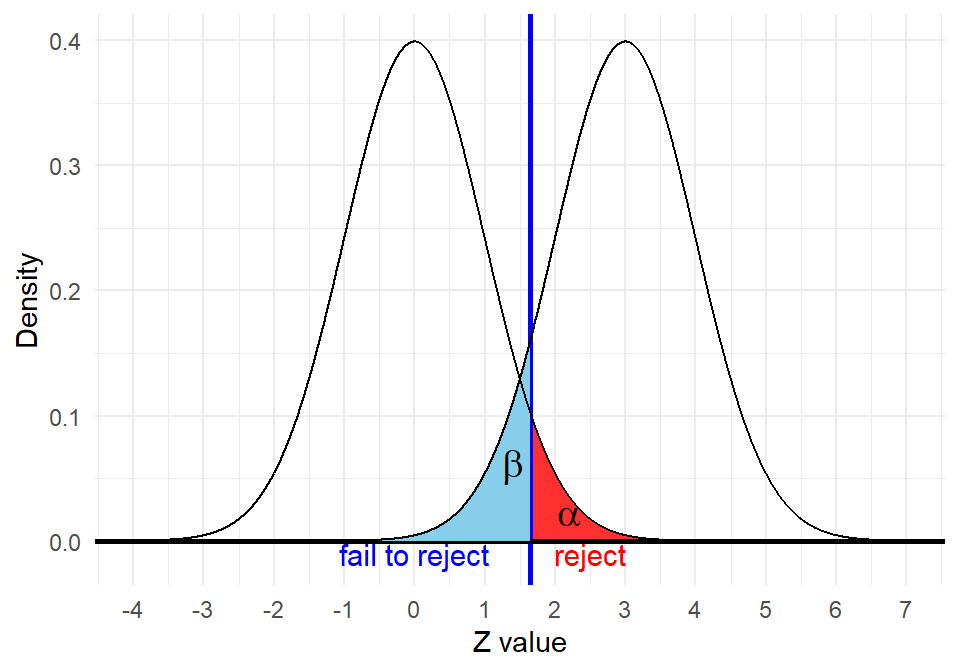# 4 Power for tests using the t distributon: Intro to the Non-central t distribution

When the standard normal (“Z”) distribution is not used for NHST tests other distributions such as the t, F and Chi-squared are often employed. A nice attribute of Z tests is that under Alternative hypotheses, sampling distributions of tests statistics such as ZM are also normal. This leads to the ability to evaluate Type II error rates and power using the standard normal distribution as we saw above. Other tests, such as “t-tests” do not have this characteristic of alternative hypothesis sampling distributions. The typical t-distributions that we have considered can be called central t-distributions. But when the null is false, not only are the sampling distributions shifted from a mean of zero, they also change shape. This shape change, in the case of t-tests, is known to follow what are called non-central t-distributions and are skewed. This section introduces non-central t-distributions using a simulation approach that parallels what was done above for the ZM test. First, we will simulate the null hypothesis sampling distribution and show that it follows the expected t distribution. Then we will simmulate the test statistic’s sampling distribution when the null is false in order to introduce the non-central t-distribution. Initially these examples are done with the same sampling situation as above, the one-sample test of a mean.

## 4.1 Visualizing the test statistic distribution for a One-sample t-test of a mean. Part I: Null hypothesis true

When evaluating a null hypothesis regarding the value of a single population mean, we reviewed (above) Type II errors and Power for the situation where the population variance is known. This leads to use of the ZM test. Now we evaluate the one-sample t-test for situations where the population variance is not known and must be estimated from the sample variance and standard deviation. The test statistic was developed previously and is

$\frac{\bar{X}-{{\mu }_{0}}}{{{{s}_{x}}}/{\sqrt{n}}\;}$.

This expression mimics the ZM construction except for the use of the sample standard deviation in the denominator. This means that each test statistic (in repeated samples) would be expected to vary in both numerator and denominator - each of the two sample statistics coming from its own sampling distribution - independently if the RV is normally distributed. This has implications for the shape of the sampling distribution of the test statistic, as we will see with the simulations below.

The approach to simulation of multiple samples is also different than how it was done above for the ZM test. The R code for creating a large number of simulated replicate samples, each with their own mean and standard deviation, is more involved. For each replicate sample, N scores are sampled from a normal distribution and from those scores, the sample mean, the sample standard deviation, the one-sample test statistic ($$\frac{\bar{X}-{{\mu }_{0}}}{{{{s}_{x}}}/{\sqrt{n}}\;}$$) and the p-value for that test are all computed.

We begin by assuming that the null hypothesis is true: $${{H}_{0}}:\mu ={{\mu }_{0}}$$ In addition we will duplicate the characteristics of the ZM test above: $${{\mu }_{0}}=100\,\,\,\,and\,\,\,{{\sigma }_{X}}=15$$ In order to do the simulation the population variance (or sd) has to be specified so that the n scores can be randomly simulated. But in an actual application of this test, these parameters are unknown and estimated from the sample data. So, the sample standard deviation is found in each replicate simulation, along with the sample mean.

The initial code chunk here creates 10000 replicate samples, each of n=25. It returns the means, sd’s, t values, and p values for each of the samples in vectors that are used in following code chunks. The ‘headTail’ function from the psych package returns the first and last four values of the 10000 replicate samples for the sample mean and t-values as an illustration of success of the simulation.

# establish basic characteristics of the sampling situation
n <- 25      # sample size
mu <- 100  # true mean of the population distribution of X (null is true)
sigma <- 15  # true SD of the population distribution of X
mu0 <- 100   # mean under the null hypothesis
reps <- 10000 # number of simulations

## initialize a few vectors:
#xvals <- as.matrix(NA,ncol=n,nrows=reps)
xbars <- numeric(reps)
stdevs <- numeric(reps)
tvals <- numeric(reps)
pvalues <- numeric(reps)

set.seed(14) # for reproducibility
for (i in 1:reps) {
x <- rnorm(n, mu, sigma)
xbars[i] <- mean(x)
stdevs[i] <- sd(x)
tvals[i] <- (mean(x) - mu0)/(sd(x)/sqrt(n))
pvalues[i] <- 2*(1 - pt(abs(tvals[i]), n-1))
# alternatively: pvalues[i] <- t.test(x, mu = mu0)$p.value } headTail(round(xbars, 2)) # round to two decimal places ## [,1] [,2] [,3] [,4] ## h "107.81" "98.31" "98.27" "98.2" ## "... ..." "... ..." "... ..." "... ..." ## t "101.39" "105.62" "100.65" "104.8" headTail(round(tvals, 2)) # round to two decimal places ## [,1] [,2] [,3] [,4] ## h "2.96" "-0.65" "-0.63" "-0.72" ## "... ..." "... ..." "... ..." "... ..." ## t "0.43" "1.96" "0.24" "1.72" Since the simulation sampled values from a normal distribution with a mean of 100, this simulated sampling distribution of $$\bar{X}$$ should also center on 100 and its shape should be normal. The ‘describe’ function shows that the mean of the simulated sampling distribution is close to 100, its standard deviation is close to the value of 3 expected ($${}^{{{\sigma }_{X}}}/{}_{\sqrt{n}}$$). Note that the skewness is close to zero, as expected for the sampling distribution of a mean based on sizeable n. #hist(pvalues,breaks=100,prob=T) #lines(density(pvalues), col="red") hist(xbars, breaks=100, prob=TRUE) lines(density(xbars, bw=.6), col="red")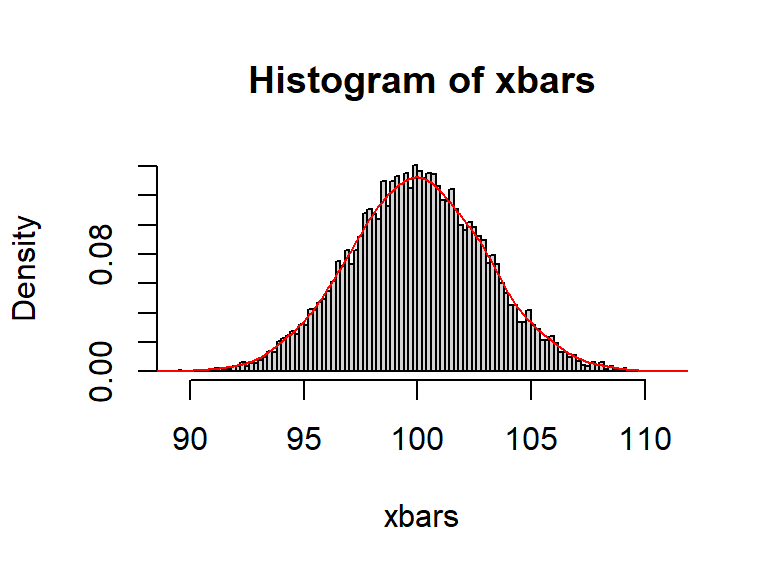d1 <- describe(xbars) d1b <- d1[,c(3,4,11)] gt(d1b) mean sd skew 99.99266 2.984081 0.01455882 Next we can examine the simulated sampling distribution of the t test statistic. It has the expected center near zero, and should look like a t distribution with df=24. Theoretically, it should also be symmetrical and we see that the skewness is close to zero hist(tvals, breaks=100, prob=TRUE) lines(density(tvals, bw=.3), col="red")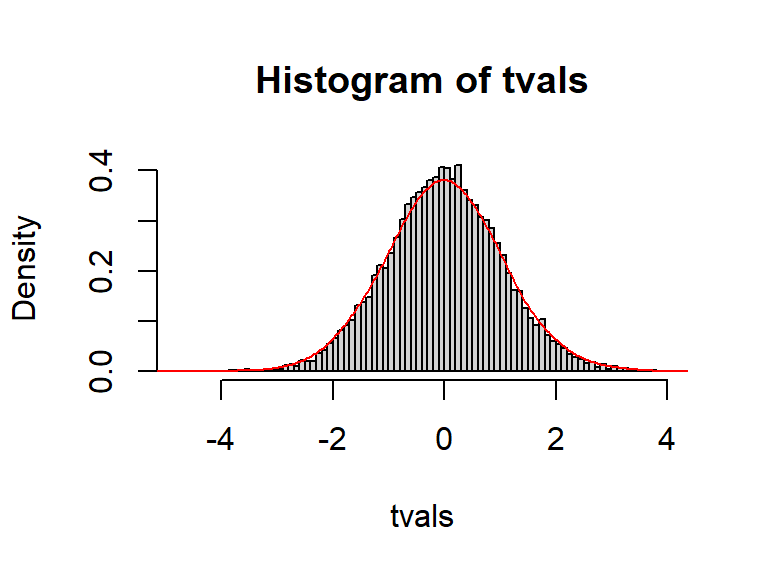d2 <- describe(tvals) d2b <- d2[,c(3,4,11)] gt(d2b) mean sd skew -0.0037544 1.0398 0.009814452 A better way to evaluate the simulated sampling distribution of the t values is to submit those values to a qq plot. Although we have only examined qqnormal plots in prior work, it is possible to compare a variable to any probability distribution. The ‘qqPlot’ function permits this. First, in this next code chunk, we look at the empirical 5th and 95th percentile values. They are close to the exact values found with the ‘qt’ function for an actual t distribution with df=24. In addition, the qq plot verifies a pretty good match of the 100000 simulated values with the theoretical t distribution. It is a little bit off in the extremeties of the tails, so even 10000 replicates generates a few outliers. Changing the seed for random number generation would modify this outlier behavior and increasing the number of replicates would minimize them. Thus when the null is true, the t test statistic behaves as expected. Also note that the 1.710882 value would be the critical value establishing the rejection region in a one-tailed t-test with df=24 and $$\alpha = .05$$. quantile(tvals, prob=c(.05,.95)) ## 5% 95% ## -1.708343 1.723015 qt(c(.05,.95), df=24) ##  -1.710882 1.710882 qqPlot(tvals,distribution="t",df=24) # qqPlot also returns the case numbers of the extreme cases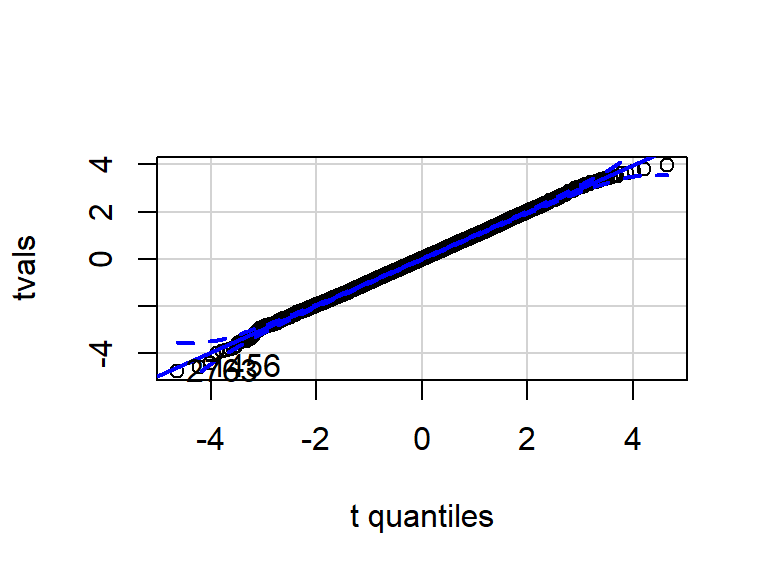##  2763 1456 Also note that the 1.710882 value seen just above would be the critical value establishing the rejection region in a one-tailed t-test with df=24, as visualized here. # library(ggfortify) p <- ggdistribution(dt, seq(-6, 6, .002), df=24, fill = 'honeydew3') p + geom_vline(aes(xintercept=qt(.05,df=24,lower.tail=F)), linetype="solid", size=1, colour="blue") + theme_minimal()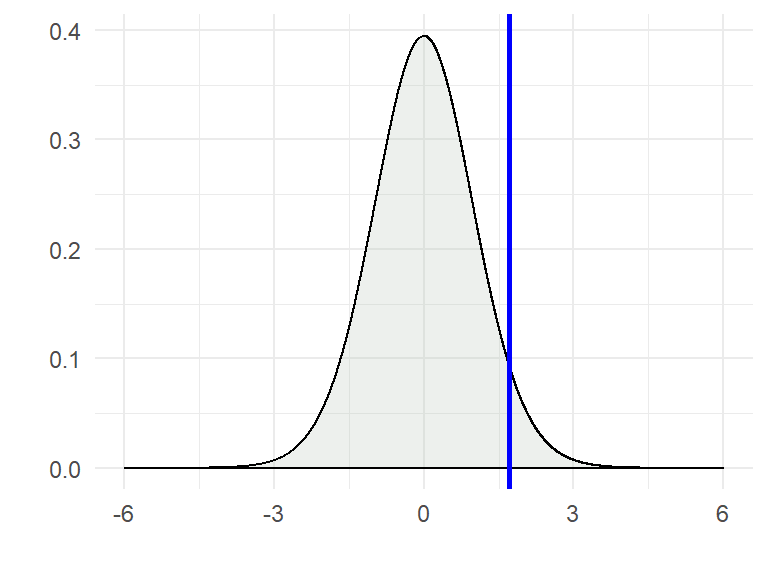## 4.2 Visualizing the test statistic distribution for a One-sample t-test of a mean. Part II: Null hypothesis False Next we will repeat the same type of simulation of the one-sample t-test, assuming that the null is false. For our purposes here let’s use the same alternative population mean that we did in the ZM simulation above ($${{\mu }_{1}}=109$$). Note that 109 is three standard errors above the null hypothesis mean of 100 (recalling that the theoretical standard error is coincidentally also 3). # establish basic characteristics of the sampling situation n <- 25 # sample size mu <- 109 # true mean of the population distribution of X (null is true) sigma <- 15 # true SD of the population distribution of X mu0 <- 100 # mean under the null hypothesis reps <- 10000 # number of simulations ## initialize a few vectors: #xvals <- as.matrix(NA,ncol=n,nrows=reps) xbars <- numeric(reps) stdevs <- numeric(reps) tvals <- numeric(reps) pvalues <- numeric(reps) set.seed(14) # for reproducibility for (i in 1:reps) { x <- rnorm(n, mu, sigma) xbars[i] <- mean(x) stdevs[i] <- sd(x) tvals[i] <- (mean(x) - mu0)/(sd(x)/sqrt(n)) pvalues[i] <- 2*(1 - pt(abs(tvals[i]), n-1)) # alternatively: pvalues[i] <- t.test(x, mu = mu0)$p.value
}
headTail(round(xbars, 2)) # round to two decimal places
##   [,1]            [,2]            [,3]            [,4]
## h "116.81"        "107.31"        "107.27"        "107.2"
##   "...       ..." "...       ..." "...       ..." "...       ..."
## t "110.39"        "114.62"        "109.65"        "113.8"
headTail(round(tvals, 2)) # round to two decimal places
##   [,1]            [,2]            [,3]            [,4]
## h "6.37"          "2.79"          "2.66"          "2.87"
##   "...       ..." "...       ..." "...       ..." "...       ..."
## t "3.18"          "5.1"           "3.5"           "4.95"

We would no longer expect the simulated sampling distribution of the mean to be centered at 100. It is close to the expected value of 109, and still normal in shape. It also has the expected standard deviation of 3.

#hist(pvalues,breaks=100,prob=T)
#lines(density(pvalues), col="red")
hist(xbars, breaks=100, prob=TRUE)
lines(density(xbars, bw=.6), col="red")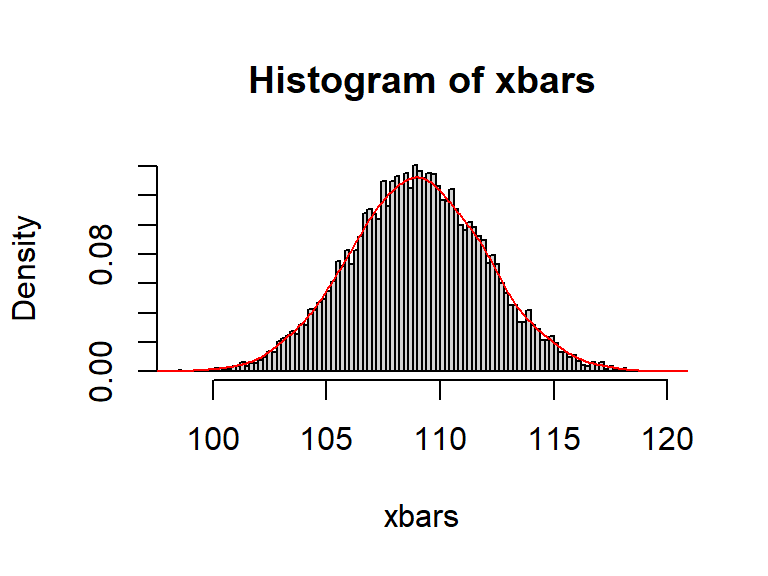d1 <- describe(xbars)
d1b <- d1[,c(3,4,11)]
gt(d1b)
mean sd skew
108.9927 2.984081 0.01455882

Next we examine the simulated sampling distribution of the t statistics. They should be located at about 3 standard errors above the null value of 100 as discussed above, and they are (mean is about 3). But a close examination of the plot and results here suggests that the simulated sampling distribution is no longer symmetrical. Some positive skewness can be seen in the histogram/density plot and the skewness coefficient is a positive value somewhat deviant from zero.

hist(tvals, breaks=100, prob=TRUE)
lines(density(tvals, bw=.3), col="red")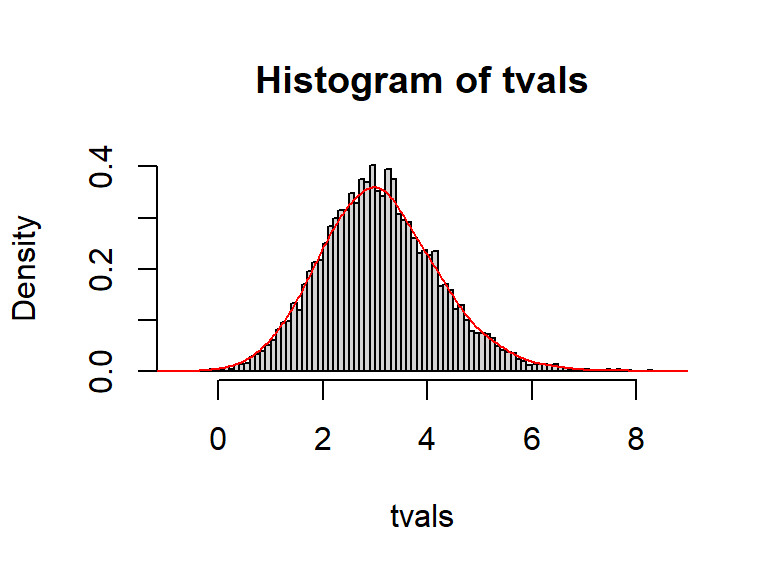d2 <- describe(tvals)
d2b <- d2[,c(3,4,11)]
gt(d2b)
mean sd skew
3.094814 1.136048 0.4008286

The positive skewness of this simulated sampling distribution can also be revealed with the qqplot. The somewhat concave of the 10000 points in the plot reflects this skewness.

qqPlot(tvals,distribution="t",df=24) # qqPlot also returns the case numbers of the extreme cases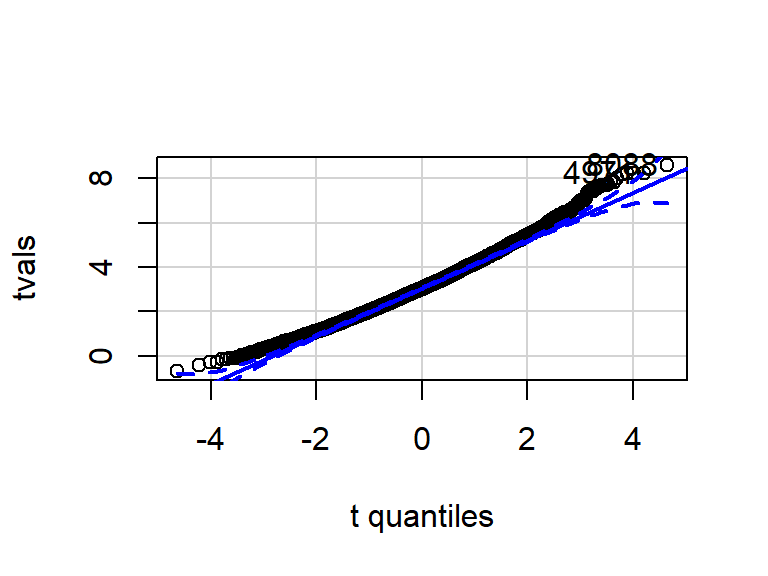##  8088 4971

At this point, we can conclude that the sampling distribution of the test statistic behaves differently under the alternative than under the null. This is not simply a matter of a shift in its location, but it’s shape is no longer symmetrical and thus does not follow a t distribution. Since Type II errors and power are found as regions of the alternative distribution, their calculation is problematic if there is not a knowable probability distribution that guides their calculation. But before taking that step, lets change a couple of aspects of the sampling simulation so that the asymmetry of the distribution is more clear.

The degree of positive skewness depends on a two characteristics, one of which is the df in the situation. So in this next simulation we will set n=9 rather than the 25 used above; df is now 8.

In order to make the arithmetic more direct/visible, another aspect of the initial population distribution of the RV (X) is also changed. Lets establish the population standard deviation ($${{\sigma }_{X}}$$) as equal to 12.0, keeping the null hypothesis mean at 100. Let’s also establish the alternative hypothesis mean as 112. With these characteristics, we would expect the sampling distribution of the t test statistic (under the alternative) to center at about 3.0 since 112 is 3 standard errors above 100 (the std error of the mean is now 12/3 or 4.0). This shift of 3 units is what we will call the non-centrality parameter, described in more detail below. The greek letter lambda ($$\lambda$$) is often used for this quantity. The value of lambda is the second characteristic that determines the degree of skewness of the distribution.

# establish basic characteristics of the sampling situation
n <- 9      # sample size
mu <- 112  # true mean of the population distribution of X (null is true)
sigma <- 12  # true SD of the population distribution of X
mu0 <- 100   # mean under the null hypothesis
reps <- 10000 # number of simulations

## initialize a few vectors:
#xvals <- as.matrix(NA,ncol=n,nrows=reps)
xbars <- numeric(reps)
stdevs <- numeric(reps)
tvals <- numeric(reps)
pvalues <- numeric(reps)

set.seed(111) # for reproducibility
for (i in 1:reps) {
x <- rnorm(n, mu, sigma)
xbars[i] <- mean(x)
stdevs[i] <- sd(x)
tvals[i] <- (mean(x) - mu0)/(sd(x)/sqrt(n))
pvalues[i] <- 2*(1 - pt(abs(tvals[i]), n-1))
# alternatively: pvalues[i] <- t.test(x, mu = mu0)\$p.value
}
headTail(round(tvals, 2)) # round to two decimal places
##   [,1]            [,2]            [,3]            [,4]
## h "1.13"          "3.14"          "3.18"          "0.91"
##   "...       ..." "...       ..." "...       ..." "...       ..."
## t "5.27"          "1.88"          "3.32"          "2.21"
hist(tvals, breaks=100, prob=TRUE, xlim=c(0,18))
lines(density(tvals, bw=.3), col="red")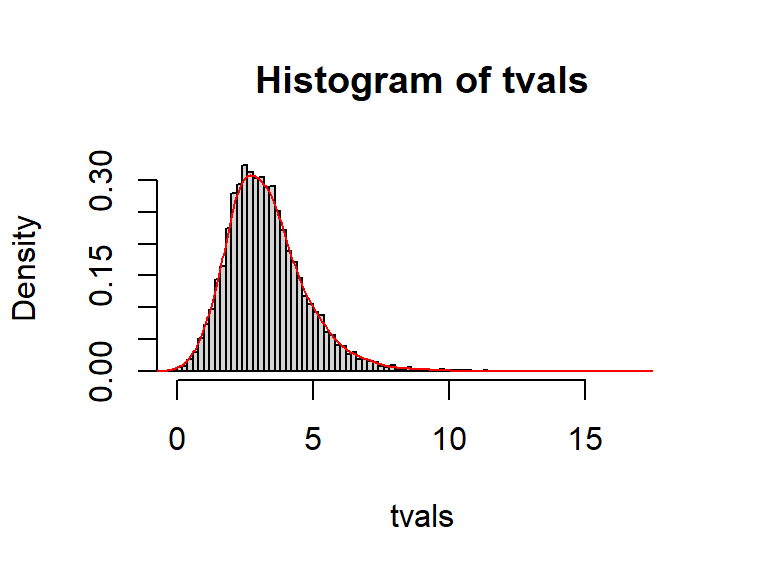d2 <- describe(tvals)
d2b <- d2[,c(3,4,11)]
gt(d2b)
mean sd skew
3.304349 1.479758 1.198309

The degree of skewness is now more visible in the histogram/density plot, and the skewness coefficient for our simulated distribution is over 1.0. Notice that the distribution is shifted above where it was (zero) for the sampling situation when the null was specified as true. It is close to the value of 3 discussed above as what the shift was expected to be. Below, there is a discussion of why the mean of this empirical sampling distribution of the t statistic is not closer to the expecte 3.0, but for now lets assume that it is close enough.

This positively skewed empirical sampling distribution is actually best described as a non-central t distribution. The t distribution centered on zero is symmetrical for all df and it is called the central t distribution. We now see that the original family of t distributions that we have introduced previously can be called central t distributions. The characteristics of non-central t’s are well understood, but for our purposes, the two primary characteristcs are lambda ($$\lambda$$), the non-centrality parameter and the degree of skewness. The non-centrality parameter is the degree of shift that the non-central t moves away from the zero value that is the center of the central t distribution, for those same df. In our example, we expected the shift to be 3 units since 112 was 3 standard errors above the null value of 100, thus $$\lambda =3.00$$.

### 4.2.1 Visualize Non-central t distributions

We can view several non-central t distributions at once using the ggdistribution function and compare to the central t distribtion. In this visualization, the df is kept at the same value as our simulation, df=8.

p <- ggdistribution(dt, seq(-7, 30, .002),df=8,ncp=0,fill = 'orange', alpha=.1) # the central t
p2 <- ggdistribution(dt, seq(-7, 30, .002),df=8,ncp=2,fill = 'honeydew2',p=p, alpha=.5)
p3 <- ggdistribution(dt, seq(-7, 30, .002),df=8,ncp=3,fill = 'honeydew2',p=p2, alpha=.5)
p4 <- ggdistribution(dt, seq(-7, 30, .002),df=8,ncp=7,fill = 'honeydew2',p=p3, alpha=.5)
p5 <- ggdistribution(dt, seq(-7, 30, .002),df=8,ncp=12,fill = 'honeydew2',p=p4, alpha=.5)
p5 +  annotate("text", label = "ncp=2", x = 3.4, y = .36, size = 4, colour = "black") +
annotate("text", label = "ncp=3", x = 4.5, y = .32, size = 4, colour = "black") +
annotate("text", label = "ncp=7", x = 8.6, y = .2, size = 4, colour = "black") +
annotate("text", label = "ncp=12", x = 14, y = .13, size = 4, colour = "black") +
annotate("text", label = "ncp=0", x = 2, y = .39, size = 4, colour = "black") +
annotate("text", label = "df=8 for all distributions", x = 22, y = .3, size = 4, colour = "black") +
labs(title="Central and Non-central t distributions")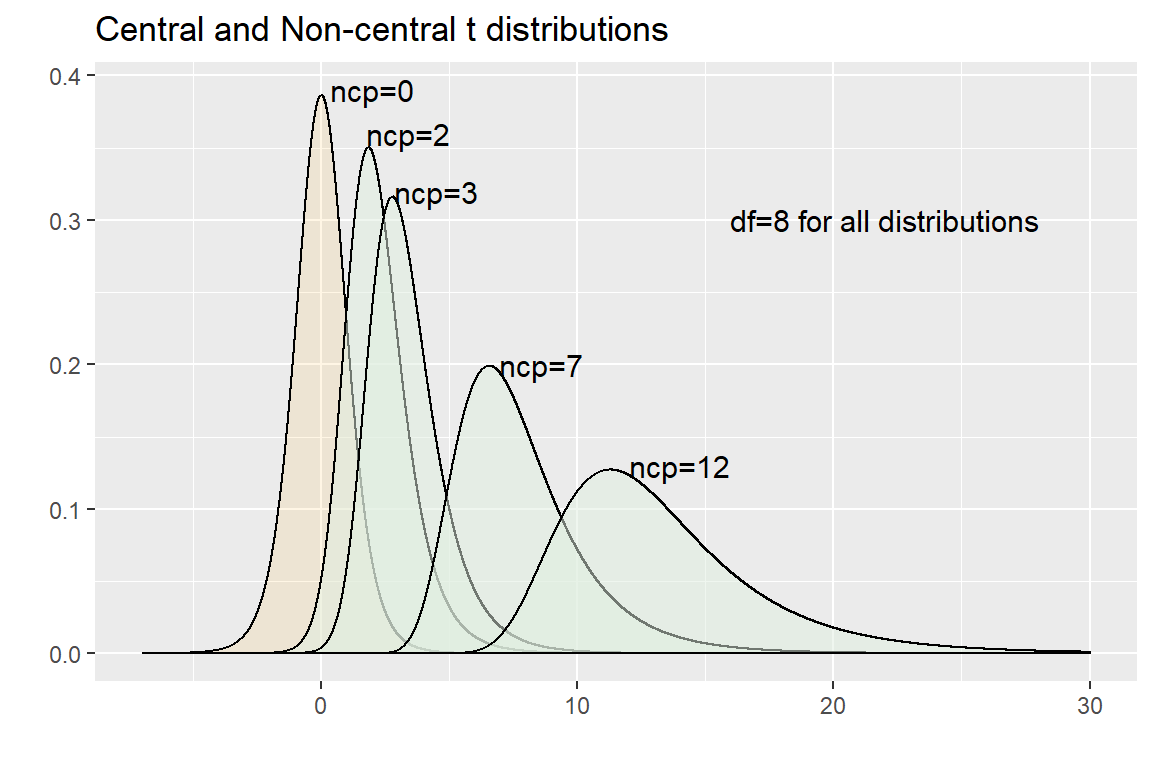Intuitively, the mean of any non-central t would be expected to be equal to the non-centrality parameter. But our simulation showed that the mean of our 10000 simulated t values, under the alternative of 112, was slightly above 3.00. This characteristic of non-central t’s is known. The larger the df, the closer the mean of a non-central t will be to $$\lambda$$. For small df, the discrepancy can be substantial. Hogben, et al (1961) have derived the exact value of moments of a non-central t distribution for varying df. For our 8 df model, a correction factor of 1.10 can be applied. Multiplying the lambda by this correction (3*1.10778) gives a value of 3.32 which is very close to the mean of our simulated sampling distribution of the t statistic under the alternative as calculated above.

Finally, realize that the example/figure here only depicted non-central t distributions where $$\lambda$$ was positive. This is because our illustration used a directional alternative in the upper tail, and thus was a one-tailed test/question. Alternatives where the mean can be lower than the null hypothesis mean would lead to $$\lambda$$ values that would be negative and the skewness of those non-central t’s would be negative, as illustrated here:

p <- ggdistribution(dt, seq(-12, 7, .002),df=8,ncp=0,fill = 'orange', alpha=.1) # the central t
p2 <- ggdistribution(dt, seq(-12, 7, .002),df=8,ncp=-3,fill = 'honeydew2',p=p, alpha=.5)
p2 +  annotate("text", label = "ncp = -3", x = -4.3, y = .31, size = 4, colour = "black") +
annotate("text", label = "ncp=0", x =1.1, y = .38, size = 4, colour = "black") +
annotate("text", label = "df=8 for both distributions", x = -9, y = .37, size = 4, colour =               "black") +
labs(title="Central and Non-central t distributions")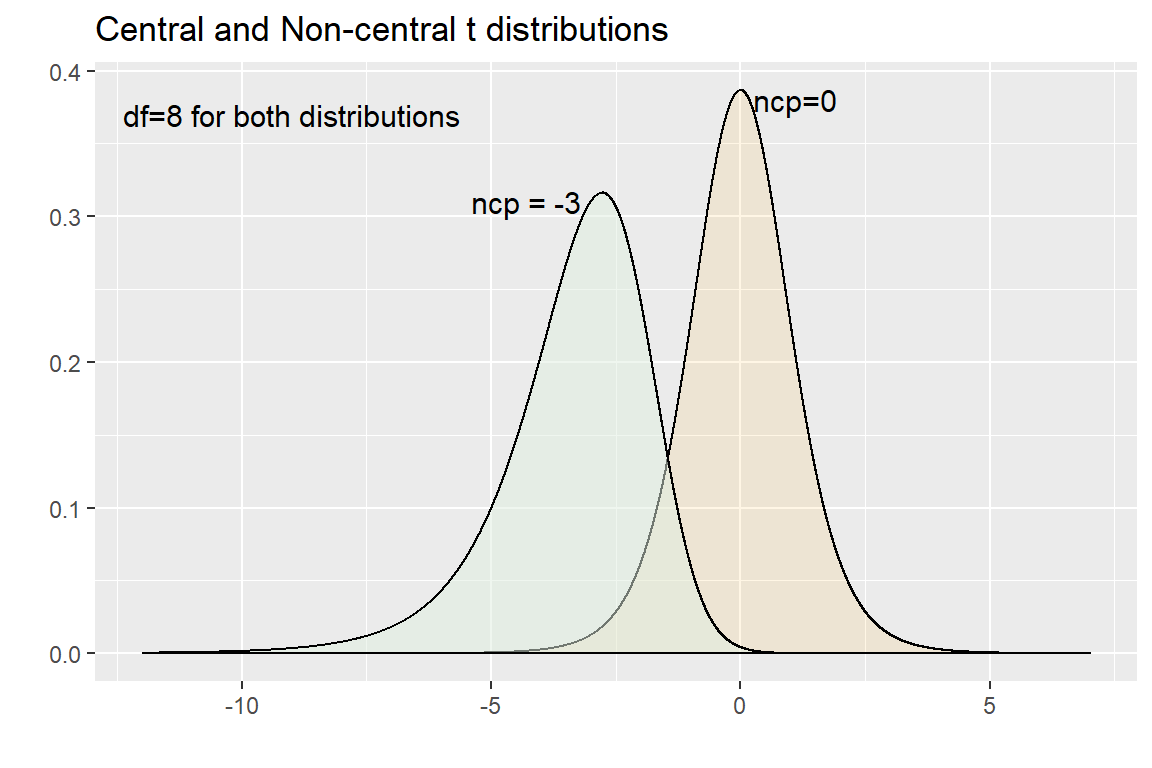## 4.3 The non-central t as a probability distribution.

The purpose of this simulation and the introduction of the non-central t distribution was to find a way to understand Type II error and power concepts in this one sample test of a mean when the population variance is not known. In order to do this, we can use R’s capability to provide probability information on non-central t’s. We can use the same suite of probability functions that we have already used with the t distribution (pt and qt). We just need to add an argument that specifies $$\lambda$$ (the argument is called ncp). For example, to find the quantile that specifies the upper 5% of a non-central t, we can use qt. Use the non-central t visualizations above (with positive ncp values) to compare (the ncp=12 distribution is the one farthest to the right in the plot with positive values of $$\lambda$$ shown above). The qt function can take an argument for the non-centrality parameter and otherwise is used as we have previously. This calculated value appears to be consistent with the visulatization - about five percent of the non-central t curve (ncp=12) sits above 20.83.

qt(.05, df=8, ncp=12,lower.tail=F)
##  20.82887

And finding the probability of a (lower tail) region with pt works analogously.

pt(9,df=8,ncp=12,lower.tail=T)
##  0.0931195

## 4.4 Visualization of Type II error regions with the non-central t distribution in the df=8 example

We can now take the final step and find the expected Type II error rate in our simulated situation. For a one-sample test of the mean, with a one-tailed test in the upper region, we specified:

$${{H}_{0}}:\mu =100$$

$${{H}_{1}}:\mu =112$$

$${{\sigma }_{X}}=12$$

n=9

and let’s set $$\alpha =.05$$

By overlaying the non-central t with the central t, and adding the location of the critical value establishing the region of rejection, we can visualize $$\beta$$ (the Type II error rate) as an area under the non-central t distribution (the blue area). This plot is the familiar way of simulultaneously viewing Type I and II error regions in the one-tailed test.

x <- seq(-5, 11, .002)
y0 <- dt(x,df=8)
y1 <- dt(x,df=8,ncp=3)
dat <- cbind.data.frame(x,y0,y1)
p3 <- ggplot(dat, aes(x = x, y = y0)) +
#geom_line() +
geom_area(mapping = aes(x = ifelse(x>qt(.05,df=8,lower.tail=F), x, 0)), fill = "firebrick1") +
xlim(-8,16) + ylim(-.01,.4) +
geom_vline(aes(xintercept=qt(.05,df=8,lower.tail=F)),
linetype="solid", size=1, colour="blue") +
#  geom_segment(aes(x = qt(.05,df=3,lower.tail=F), y = 0,
#                   xend = qt(.05,df=3,lower.tail=F), yend = .04, colour = "blue"),data=dat)
geom_hline(aes(yintercept=0),
linetype="solid", size=1, colour="black") +
annotate("text", label = "fail to reject", x = -2, y = -.01, size = 4, colour = "blue") +
annotate("text", label = "reject", x = 6.5, y = -.01, size = 4, colour = "red") +
annotate("text", label = expression(alpha), x = 2.6, y = .012, size = 5, colour = "black") +
scale_x_continuous(breaks=seq(-5,11,2)) +
labs(x="t value", y="Density") +
theme_minimal()
p3 +
geom_area(mapping = aes(x = ifelse(x<qt(.05,df=8,lower.tail=F), x, 0), y=y1), fill = "skyblue") +
geom_line(mapping=aes(x=x,y=y1)) +
geom_line(mapping=aes(x=x,y=y0)) +
annotate("text", label = expression(beta), x = 1.5, y = .08, size = 5, colour = "black")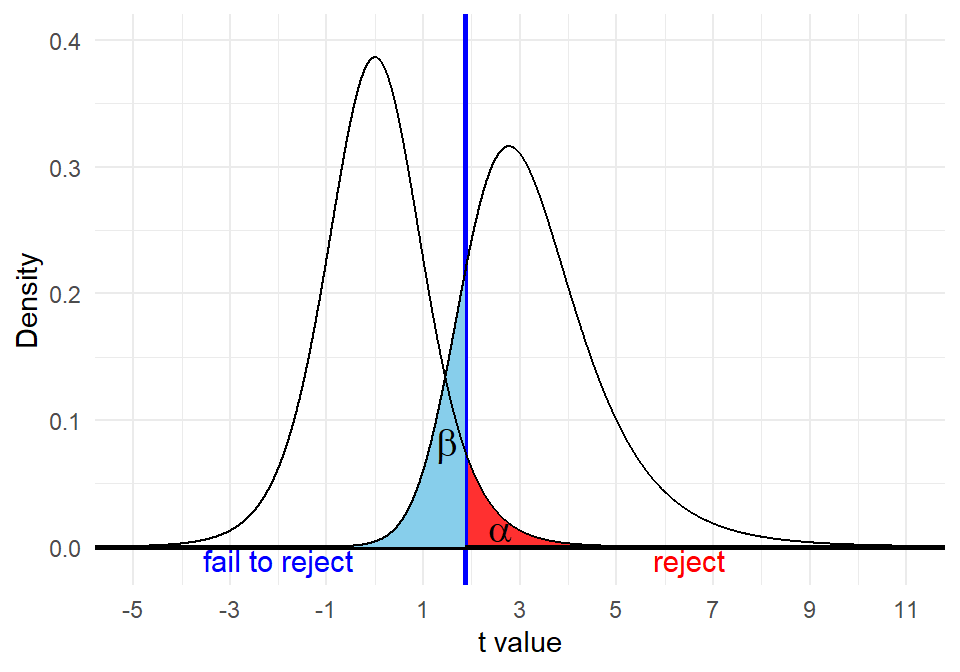We can also compute the Type II probability exactly, using the critical value that is established under the null hypothesis central t distribution. Visually, this computation looks correct - about 14% of the non-central t curve falls below the CV (the blue area).

pt(qt(.05,df=8, lower.tail=F), df=8,ncp=3, lower.tail=T)
##  0.1381863

## 4.5 Visualization of Type II error regions in the original df=24 example

We originally did ZM and one-sample t-test simulations with an n=25 sampling situation with different alternative means (109) and standard deviations (15). The switch to the smaller sample size was done so that the skewness of the non-central t simulation could be better visualized. But now we can return to the earlier example characteristics and find $$\beta$$ using the tools developed just above. With these parameters, the mean of 109 is 3 standard errors of the mean above the null value of 100. Therefore, $$\beta$$=3.00.

First, the visualization:

x <- seq(-4, 8, .002)
y0 <- dt(x,df=24)
y1 <- dt(x,df=24,ncp=3)
dat <- cbind.data.frame(x,y0,y1)
p3 <- ggplot(dat, aes(x = x, y = y0)) +
#geom_line() +
geom_area(mapping = aes(x = ifelse(x>qt(.05,df=24,lower.tail=F), x, 0)), fill = "firebrick1") +
xlim(-8,16) + ylim(-.01,.4) +
geom_vline(aes(xintercept=qt(.05,df=24,lower.tail=F)),
linetype="solid", size=1, colour="blue") +
#  geom_segment(aes(x = qt(.05,df=3,lower.tail=F), y = 0,
#                   xend = qt(.05,df=3,lower.tail=F), yend = .04, colour = "blue"),data=dat)
geom_hline(aes(yintercept=0),
linetype="solid", size=1, colour="black") +
annotate("text", label = "fail to reject", x = -1, y = -.01, size = 4, colour = "blue") +
annotate("text", label = "reject", x = 4, y = -.01, size = 4, colour = "red") +
annotate("text", label = expression(alpha), x = 2.3, y = .012, size = 5, colour = "black") +
scale_x_continuous(breaks=seq(-4,8,2)) +
labs(x="t value", y="Density") +
theme_minimal()
p3 +
geom_area(mapping = aes(x = ifelse(x<qt(.05,df=24,lower.tail=F), x, 0), y=y1), fill = "skyblue") +
geom_line(mapping=aes(x=x,y=y1)) +
geom_line(mapping=aes(x=x,y=y0)) +
annotate("text", label = expression(beta), x = 1.3, y = .05, size = 5, colour = "black")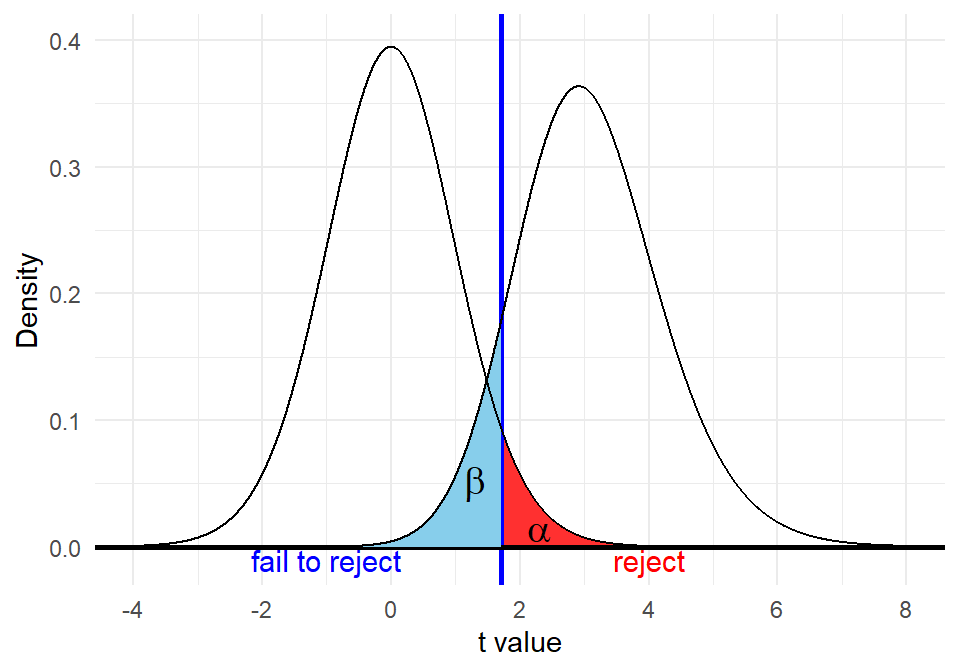The exact value of $$\beta$$ is found again with pt by passing the critical value, based on the null, as the first argument. The returned value of about .10 is visualized as the blue area in the above figure. Power in this situation would be approximately 90%.

pt(qt(.05,df=24,lower.tail=F),df=24,ncp=3, lower.tail=T)
##  0.1022361

# 5 Why is the non-central t asymmetrical?

The short, less mathematical, answer to the question of the origin of the asymmetry of the non-central t is that it derives from the fact that the t is a ratio of one RV (sample mean) to another RV (sample standard deviation) - each adjusted by a constant ($${{\mu }_{0}}$$) and n, respectively. The ZM is the ratio of a random variable (sample mean) to a constant, so its distribution would follow the shape of the RV (the sample mean) as we saw in the simulation. But for the t statistic, the numerator is normally distributed (sampling distribution of the mean) but the denominator is not. We have covered the idea that the sample variance can be seen as a Chi-square derived value and Chi-squared distributions are positively skewed (moreso for lower df). So, the sample standard deviation is also positively skewed (especially for low df) as we visualized in a shiny app. So one of the reasons for the asymmetry comes from the math of ratios of normal RV’s to non-normal RV’s. But a second reason comes from the fact that the actual mean of a non-central t is not exactly equal to the value of $$\lambda$$, and this discrepancy is larger for lower df and larger $$\lambda$$ (this was addressed above). These two things produce the skewness of the non-central t.

# 6 Non-centrality parameter, effect size and t statistic

In order to extend fully our understanding of power for purposes of sample size planning, and to incorporate the role of the non-centrality parameter, we also need to connect these concepts to the concept of an effect size. In order to accomplish this, let’s revist the non-centrality parameter more formally. Although some treatment of this is in the Howell (2013) textbook, this section is heavily influenced by Cumming and Finch (2001). In sections above, we discussed how when an alternative hypothesis is correct, that we would expect the sampling distribution of our one-sample test statistic to be shifted away from the zero-centered value of the central t distribution. The amount of this shift was described as lambda and shown with simulation. We can see this in the relevant expressions. The test statistic for the one-sample t-test is:

$\frac{\bar{X}-{{\mu }_{0}}}{{}^{{{s}_{x}}}/{}_{\sqrt{n}}}$.

When the null is true, $$\bar{X}$$ deviates above and below the null mean in such as way that the expected value of the test statistic is zero. But when an alternative hypothesis is true, $$\bar{X}$$ deviates from the specified $$\mu_{0}$$ with larger deviations and the sampling distribution of the test statistic will deviate above or below the zero value expected under the null. The amount of this shift depends on the true value of the population mean. If the true value of $$\mu$$ is equal to an alternative hypothesis mean ($$\mu_{1}$$) then we can rewrite the numerator of the test statistic by breaking the deviation into two components:

$\left( \bar{X}-{{\mu }_{1}} \right)+\left( {{\mu }_{1}}-{{\mu }_{0}} \right)$

This reflects the fact that the total deviation from the null mean is composed of a deviation of the sample mean from the true population mean plus a component that reflects the shift of the sampling distribution from a center of $$\mu_{0}$$ to a center of $$\mu_{1}$$. So the test statistic now can be rewritten to reflect this:

$\frac{\left( \bar{X}-{{\mu }_{1}} \right)+\left( {{\mu }_{1}}-{{\mu }_{0}} \right)}{^{{{s}_{x}}}{{/}_{\sqrt{n}}}}\tilde{\ }{{t}_{n-1,\lambda }}$

What this expression tells us is that our test statistic will be distributed as a non-central t with n-1 df, and with a non-centrality parameter of $$\lambda$$. The non-centrality emerges from the amount of shift described as the second phrase in the numerator $$\left( {{\mu }_{1}}-{{\mu }_{0}} \right)$$ but lambda is actually this deviation standardized relative to the true standard error in the system:

$\lambda =\frac{{{\mu }_{1}}-{{\mu }_{0}}}{^{{{\sigma }_{x}}}{{/}_{\sqrt{n}}}}$

With that simulation and formal defninitions of lambda now in place we need to review the effect size concept as the next step to using power to plan sample sizes. In prior work, we had introduced the standardized effect size statistic called Cohen’s d in the two-sample location or independent samples t-test context. There is an analogous effect size statistic for the one-sample test emphasized above throughout this document. It simply indexes the discrepancy of the observed sample mean from the null hypothesis mean relative to the variation of the variable (as a st